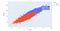# Simple Linear Regression Pt.1

I’ll start to discuss linear regression today by building a simple regression model. In the subsequent blog, We’ll unpack some of the results for a better understanding of what linear regressions are and how we can interpret the data.

Regressions are a way to model the relationship between features of a data set such that one feature is dependent upon one or more other features. For example, we would generally say there is a relationship between a person’s height and their weight, ie, people who are taller will generally weigh more than people who are shorter. Obviously, this is not always the case as you can have people who are very heavy despite being really short and comparatively light.

To examine this further, let look at some weight-height data found in a data set on Kaggle. Let's take a look.

In a python jupyter notebook, we start with some imports that I can use.

`import pandas as pdimport statsmodels.api as smfrom statsmodels.formula.api import olsimport matplotlib.pyplot as pltimport plotly.express as pximport seaborn as snsplt.style.use('seaborn')`

I’ll use pandas to connect to the CSV file with the data and read that into a data frame. Then I’ll get a glimpse of first five rows of data by calling df.head().

`df = pd.read_csv('weight-height.csv')df.head()`

Ok, I see three features in the dataframe but I’m not sure how many rows of data I have. I want to get the overall shape of the data set and get a general description as well.

`print(df.shape)df.describe()`

We can now see that our data 3 columns of data for 10 thousand rows. Of the three features, Gender is categorical data and consequently is dropped from our descriptive statistics which require numeric data. We can see above that the mean height is just over 66 inches while the mean weight is 161 pounds. Can we see a relationship between Height and Weight? We can if we visualize the data. Here I’ll build a simple scatterplot in matplotlib to determine if there are correlations between Weight and Height.

`plt.scatter(df.Height, df.Weight)plt.xlabel('Height')plt.ylabel('Weight')plt.title("Plotting Correlations")`

We can see here a very tight relationship between the two. Actually, I have to wonder if the relationship is too tight; related to the Kaggle data was a discussion questioning whether the data was simulated as it was perhaps not realistic for weights and height. I cannot say, and there is no description with the data stating how the data was gathered. So I won’t read too much into this and am using it for the purposes of working with data only.

I tend to like Plotly visualizations better than matplotlib; I find them easier to use and often the results are more attractive. With scatter plots it won’t look much different, but I like the feature that allows for popups describing a specific data point when we hover the cursor over the plot. I’ll plot it now in plotly and this time I’ll add color based on gender to get a better sense of how the data looks:

`fig = px.scatter(df, x = "Height", y= "Weight", color = "Gender",                  title = "Plotting Correlations")fig.show()`Note: The title of the Plot is “Plotting Correlations”. It couldn’t fit into the screenshot and was cut off. It is in the original notebook.

Now that’s a lot clearer and we can see the height and weight distributions for males and females clearly. Also, I left the popup where I hovered over a point: that particular individual was a male with a height of 73.6 inches (6'1") and a weight of just over 225 pounds.

In the next blog, I’ll model this data using a method called Ordinary Least Squares. Stay tuned!